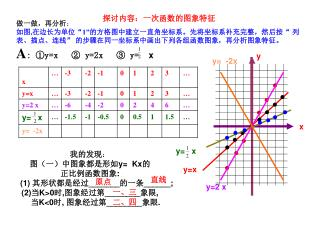DownloadDownload Presentation探讨内容：一次函数的图象特征

Download Presentation探讨内容：一次函数的图象特征

- - - - - - - - - - - - - - - - - - - - - - - - - - - E N D - - - - - - - - - - - - - - - - - - - - - - - - - - -
Presentation Transcript

1. 做一做，再分析： 如图,在边长为单位“1”的方格图中建立一直角坐标系。先将坐标系补充完整，然后按“ 列 表、描点、连线” 的步骤在同一坐标系中画出下列各组函数图象，再分析图象特征。 A: ①y=x ② y=2x ③ y=x y y= -2x ● ● ● ● ● ● ● 1 ● ● ● 2 x ● ● ● ● ● ● ● ● y= x ● y=x ● y=2 x ● 探讨内容：一次函数的图象特征 x 我的发现： 图（一）中图象都是形如y= Kx的 正比例函数图象: (1) 其形状都是经过_______的一条______; (2)当K>0时,图象经过第________象限, 当K<0时, 图象经过第________象限. 直线 原点 一、三 二、四

2. y 2 ● ● ● ● ● ● -2 0 2 ● ● -2 ● ● ● ● x ● ● ● ● ● ● ● ● ● ● ● ● ● ● y=2x+1 y=2x-1 y=2x B: ①y=2x ② y=2x+1 ③y=2x-1 我们发现: 在图（二）中, ② y=2x+1 ③y=2x-1的图象与①y=2x 的图象仅有______不同,且三 图象相互______.且由各点坐标可知, ② y=2x+1和 ③y=2x-1的图象可看成是由①y=2x的图象作________平移后得到的.即将 ①y=2x的图象向____平移_____个单位后就得到② y=2x+1的图象;将①y=2x的图象 向____ 平移_____个单位后就得到 ②y=2x-1的图象. 位置 平行 上、下 上 1 下 1

3. y y= -2x+2 y= -2x 2 ● ● y= -2x-2 ● ● ● ● ● -2 0 2 -2 ● ● ● ● x ● ● ● ● ● ● ● ● ● ● ● ● ● ● ● C: ①y=-2x ② y=-2x+2 ③y=-2x-2 我们发现: 在图（三）中, ② y=-2x+2 ③y=-2x-2 的图象与①y=-2x的图象仅有______不同, 且三图象相互______.且由各点坐标可知, ②y=-2x+2和 ③y=-2x-2的图象可看成 是由①y=-2x的图象作_____平移后得到的. 即将①y=-2x的图象向___平移___个单位 后就得到② y=-2x+2的图象;将①y=2x 的图象向____平移____个单位后就得到 ③y=-2x-2的图象. 位置 平行 上、下 上 2 下 2 你能概括出y=kx(k≠0)的图象和y=kx+b(k≠0)的图象间的联系吗?

4. y 0 x 正比例函数y=kx(k≠0)的图象和一次函数y=kx+b(k≠0)的图象间的联系： 将正比例函数y=kx(k≠0)的图象作上、下平移后就可得到一次函数y=kx+b(k≠0)的图象：（1）当b>0时,上移︱b︱个单位;(2)当b<0时,下移︱b︱个单位. 3x+4 例如:将y=3x的图象向上平移4个单位后得到y=___________的图象, 将y=3x的图象向下平移6个单位后得到y=___________的图象; 又如:将y=- 3x的图象向上平移1个单位后得到y=___________的图象; 将y=- 3x的图象向下平移6个单位后得到y=___________的图象, 且这时的图象与x轴交于点( ____ , ____ )与y轴交于点( ____ , ____ ) . 3x-6 - 3x+1 - 3x- 6 0 - 6 - 2 0 试一试 一、二、三 一、二、四 1、 y=3x+2的图象经过第____________象限， y=-2x+5的图象经过第___________象限， y=-6x-5的图象经过第___________象限， y=2x-8的图象经过第____________象限， y=-4x-1的图象不经过第_______象限， y=12x+9的图象不经过第_________象限 二、三、四 一、三、四 四 一

5. y y 0 x 0 x 2、已知一次函数y=-3x+12的图象与与x轴交于点A，与y轴交于点B，点O为坐标原点，则△ABO的面积为___________ 24 5x+2 3、将y=5x的图象向上平移2个单位后得y=___________的图象, 将y=- 3x的图象向下平移7个单位后得到y=___________的图象; - 3x-7 -4x 4、 一次函数y=-4x+8的图象可看作是由正比例函数y= _________的 图象向____平移____个单位后得到的，该图象与x轴交于点与x轴 交于点( ____ , ____ )与y轴交于点( ____ , ____ ) 上 8 2 0 0 8